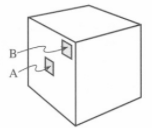# Problem: A positive charge is located at the center of a cube. a. Are the intersections of the field lines with a side of the box uniformly distributed across that side? Explain. b. We can consider the left side of the box as composed of many small surface elements of equal area. i. Is the number of field lines through surface element A greater than, less than, or equal to the number of field lines through surface element B? Explain. ii. Is the flux through surface element A greater than, less than, or equal to the flux through surface element B? Explain. c. Consider the surface element A itself as composed of many even smaller pieces. Would the number of field lines through each of those new small surface elements vary much from one to another? Explain. Describe how the field lines for the positive point charge appear to be distributed when the region over which you look becomes sufficiently small.

###### FREE Expert Solution

Electric field:

$\overline{){\mathbf{E}}{\mathbf{=}}\frac{\mathbf{k}\mathbf{q}}{{\mathbf{r}}^{\mathbf{2}}}}$, where r is the distance from the point charge q.

a.

From the electric field equation, it is clear that r varies on the same side of the cube.

99% (483 ratings)###### Problem Details

A positive charge is located at the center of a cube.a. Are the intersections of the field lines with a side of the box uniformly distributed across that side? Explain.

b. We can consider the left side of the box as composed of many small surface elements of equal area.

i. Is the number of field lines through surface element A greater than, less than, or equal to the number of field lines through surface element B? Explain.

ii. Is the flux through surface element A greater than, less than, or equal to the flux through surface element B? Explain.

c. Consider the surface element A itself as composed of many even smaller pieces. Would the number of field lines through each of those new small surface elements vary much from one to another? Explain. Describe how the field lines for the positive point charge appear to be distributed when the region over which you look becomes sufficiently small.

Frequently Asked Questions

What scientific concept do you need to know in order to solve this problem?

Our tutors have indicated that to solve this problem you will need to apply the Electric Field concept. You can view video lessons to learn Electric Field. Or if you need more Electric Field practice, you can also practice Electric Field practice problems.

What professor is this problem relevant for?

Based on our data, we think this problem is relevant for Professor De Antonio's class at NMSU.